# Homework H.5.G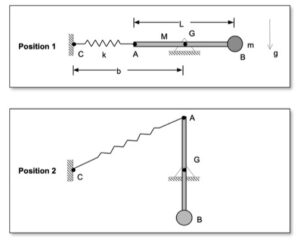Discussion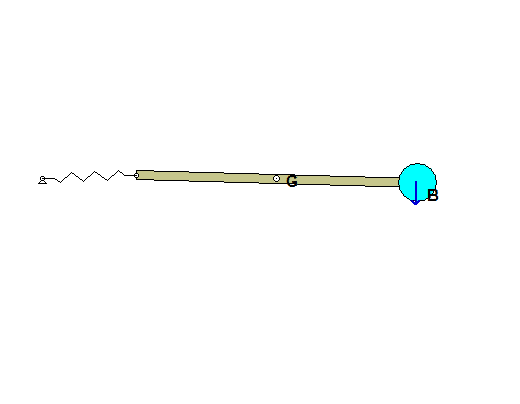The animation shown above demonstrates the kinematics of the motion of the bar/particle/spring system. The bar rotates about its center of mass G. The particle is attached to end B of the bar such that the particle has the same velocity at point B on the bar. The system is released from rest with the bar being horizontal and the spring being unstretched.

You are asked to determine the rotation rate of the bar when the bar reaches the vertical position shown below.HINT: As always, we should follow the four-step plan for solving this problem.
STEP 1: FBD. We will be using the work/energy equation to determine this angular speed. Based on earlier recommendations, we will make the choice of our system BIG, including the bar, particle and spring.
STEP 2: Kinetics (here, work/energy). The total kinetic energy of the system shown in your FBD above is that of the bar + that of the particle. The center of mass of the bar is a fixed point, making the choice of reference point for KE easy. The KE of the particle is simply 0.5*m*vB2. The spring is initially unstretched, so the spring does not contribute to the PE at position 1. Be sure to identify the datum line for the gravitational potential energy, and use this in writing down this potential.
STEP 3: Kinematics. You need to relate the speed of B to the angular speed of the bar.
STEP 4: Solve

# Homework H.5.HDiscussion
The most complicated part of this problem is its kinematics. Note that since the wheel rolls without slipping, its instant center is the contact point of the wheel with the incline (let's call that point C). The speed of any point on the wheel is proportional to the distance between that point and C. You can see this in the following animation made from the simulation of the motion of the wheel for this problem.Of particular interest is the velocity of the topmost point on the wheel where the cord comes off the perimeter of the wheel (let's call that point A). This is shown below in a freeze-frame of the wheel's motion.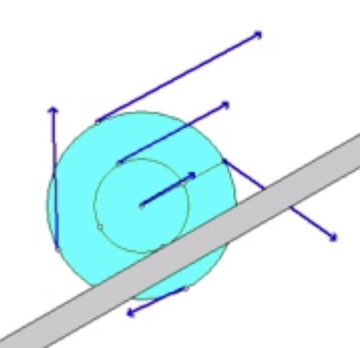From this, we can see that the speed of A is three times that of point O. Since vA = 3vO for all time, then we can conclude that the end of the cord moves three times the distance traveled by O. This is important information in calculating the work done by the force F.

As always, you need to follow the four-step plan in solving:

Step 1: Free body diagram of the wheel.
Step 2: Kinetics. Which reference point will you use for finding the kinetic energy? Also, be sure to use the above result for finding the work done by F.
Step 3: Kinematics. What is needed?
Step 4: Solve.

# Homework H.5.ENOTE: The system is released from rest.

# Homework H.5.F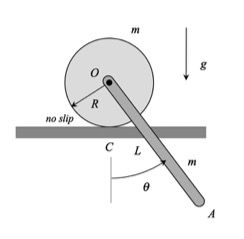DiscussionThe animation above shows the acceleration of the two centers of mass O and G for the disk and bar, respectively. As expected, the acceleration of O is always along a horizontal line. The direction of the acceleration of G is more complicated: its direction depends on the angle of the bar as well as on the angular velocity of the bar and the acceleration of point O. You will deal with this is STEP 3 below.

If we freeze-frame the animation at the time of release from rest, we see the directions of the acceleration of points O and G, as shown below.HINT: As always, we should follow the four-step plan for solving this problem.

STEP 1: FBDs (draw INDIVIDUAL FBDs of the bar and disk)
STEP 2: Kinetics (here, Newton-Euler) Be careful on your choice of reference point in Euler's equation for the bar. For example, you cannot use point O as your reference point in using the short form of Euler's equation; that is, MO ≠ IO* αbar.
STEP 3: Kinematics. Here, you will need two acceleration kinematics equations. One that relates the acceleration of C to the acceleration of O, and a second that relates the accelerations of O and G (the cm of the bar). Be careful to give the angular accelerations of the disk and bar distinct names so as to not confuse them as you solve your equations. The angular acceleration of the bar is NOT equal to the angular acceleration of the disk. This is easily seen in the animation above.
STEP 4: Solve

# Homework H.5.CNOTE: The view of the figure provided is that of the rod as seen from above the surface on which the rod lies.

# Homework H.5.DDiscussionThe animation shown above demonstrates the kinematics of the motion of the bar. (Note that in the animation velocities are shown in BLUE and accelerations are shown in RED.) In particular, we are reminded from this that:

• End A moves along a straight line path dictated by the angled wall. Since the path of A is straight, the acceleration of A is always aligned with the wall surface.
• End B moves on a circular path centered on point O. Here, since the path of B is curved, the acceleration of B will have, in general, two components of acceleration: one component that is tangent to the path of B and the second component that is normal to the path of B. This second component is the centripetal component of acceleration, and is given by aBn = vB2/R, where R is the length of rod OB. Since the bar is released from rest, vB = 0; therefore, the normal component of the acceleration for B is zero. As a result, the acceleration of B is tangent to the path of B( that is, perpendicular to OB) on release.

The above kinematics observations are seen if we freeze-frame the above animation at the time that it is released from rest. See below. Here we see the acceleration of A is down along the slot, and the acceleration of B is horizontal.HINT: As always, we should follow the four-step plan for solving this problem.
STEP 1: FBD
STEP 2: Kinetics (here, Newton-Euler)
STEP 3: Kinematics. See discussion of this above. You will need to use the known directions of the accelerations of A and B in order to write down the acceleration of G needed for Newton's equation. Click on this link to see how this is done. See Example 5.A.13 of the lecture book as an example on how to approach the kinematics of a problem such as this with constrained motion for two points on a rigid body.
STEP 4: Solve

# Homework H.5.A# Homework H.5.BNOTE: The system moves in a horizontal plane.

# Homework H.4.S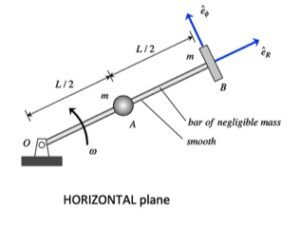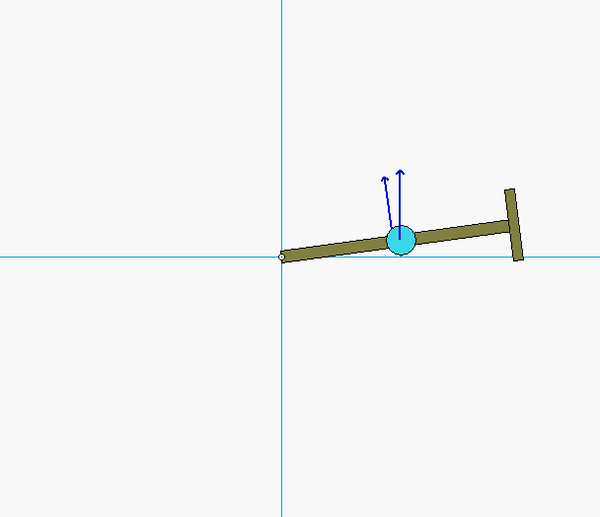You are asked to investigate the dynamics of this system during the time from releases through to the time of immediately after A strikes B.

• Divide this time of investigation into two segments: 1 to 2, and 2 to 3.
• From times 1 to 2, A slides outward on the arm until it almost impacts B. During this time, angular momentum of A+B+arm  is conserved, as well an energy for that same system is conserved.
• From times 2 to 3, A impacts B. During this time, angular momentum of A+B+arm is conserved; however, due to the impact, energy is NOT conserved. Instead, we will use the coefficient of restitution as the second equation.

HINTS:

STEP 1 - FBD: Draw a SINGLE free body diagram (FBD) of the system of A+P+arm.
STEP 2 - Kinetics: The only force that you will see acting on this system during the full range of time from 1 to 3 is the support force at O. Because this forces acting through point O, angular momentum  about O is conserved throughout. During the pre-impact time of 1 to 2, also write down a conservation of energy equation. During the impact time of 2 to 3, write down the COR equation involving only the radial components of velocity.
STEP 3 - Kinematics: At Instant 1, A and B have only eθ components of velocity. For Instants 2 and 3, A has both eand eθ components of velocity, and B has only eθ components of velocity.
STEP 4 - Solve.

# Homework H.4.TDiscussion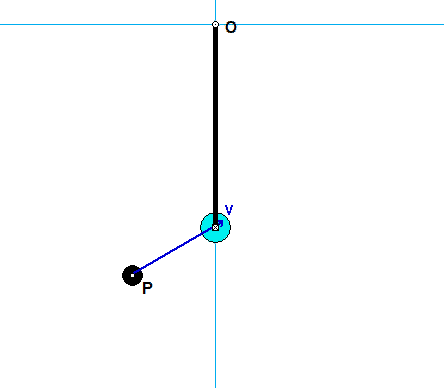You are asked to investigate the dynamics of this system during the short time of impact of P with A.

• It is suggested that you consider a system made up of A+P+bar (make the system "big").
• Draw a free body diagram (FBD) of this system.
• For this system, linear momentum is NOT conserved since there are non-zero reaction forces at O.
• Furthermore, energy is NOT conserved since there is an impact of P with A during that time.
• From your FBD of the system, you see that the moment about the fixed point O is zero. What does this say about the angular momentum of the system about O during impact? (Answer: It is conserved!)

HINTS:

STEP 1 - FBD: Draw a SINGLE free body diagram (FBD) of the system of A+P+bar.
STEP 2 - Kinetics:  Consider the discussion above in regard to conservation of angular momentum about point O. Recall how to calculate the angular momentum about a point for a particle.
STEP 3 - Kinematics: At Instant 2, the P sticks to A: vP2 = vA2.
STEP 4 - Solve.